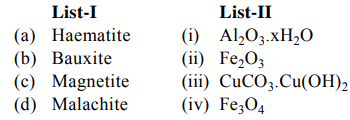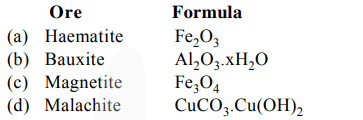# Match List-I and List-II :`
Question:

Match List-I and List-II :.Choose the correct answer from the options given below:

1. $(\mathrm{a})-(\mathrm{ii}),(\mathrm{b})-(\mathrm{iii}),(\mathrm{c})-(\mathrm{i}),(\mathrm{d})-(\mathrm{iv})$

2. (a)-(iv), (b)-(i), (c)-(ii), (d)-(iii)

3. (a)-(i), (b)-(iii), (c)-(ii), (d)-(iv)

4. (a)-(ii), (b)-(i), (c)-(iv), (d)-(iii)

Correct Option: , 4

Solution: